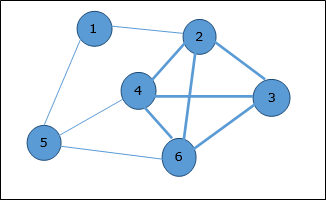• Design and Analysis of Algorithms
• Home

Design and Analysis Max Cliques

In an undirected graph, a clique is a complete sub-graph of the given graph. Complete sub-graph means, all the vertices of this sub-graph is connected to all other vertices of this sub-graph.

The Max-Clique problem is the computational problem of finding maximum clique of the graph. Max clique is used in many real-world problems.

Let us consider a social networking application, where vertices represent people’s profile and the edges represent mutual acquaintance in a graph. In this graph, a clique represents a subset of people who all know each other.

To find a maximum clique, one can systematically inspect all subsets, but this sort of brute-force search is too time-consuming for networks comprising more than a few dozen vertices.

Algorithm: Max-Clique (G, n, k)
S := Φ
for i = 1 to k do
t := choice (1…n)
if t Є S then
return failure
S := S ∪ t
for all pairs (i, j) such that i Є S and j Є S and i ≠ j do
if (i, j) is not a edge of the graph then
return failure
return success

Analysis

Max-Clique problem is a non-deterministic algorithm. In this algorithm, first we try to determine a set of k distinct vertices and then we try to test whether these vertices form a complete graph.

There is no polynomial time deterministic algorithm to solve this problem. This problem is NP-Complete.

Example

Take a look at the following graph. Here, the sub-graph containing vertices 2, 3, 4 and 6 forms a complete graph. Hence, this sub-graph is a clique. As this is the maximum complete sub-graph of the provided graph, it’s a 4-Clique.Useful Video Courses

Video

Compiler Design Online Training

102 Lectures 10 hours

Video

Sequential Circuit Design

30 Lectures 3 hours

Video

Design Patterns Online Training

31 Lectures 4 hours

Video

InDesign Online Training

43 Lectures 1.5 hours

Video

Canva: Become a Graphic Designer

7 Lectures 1 hours

Video

The Ultimate Canva Graphic Design Course

54 Lectures 4 hours